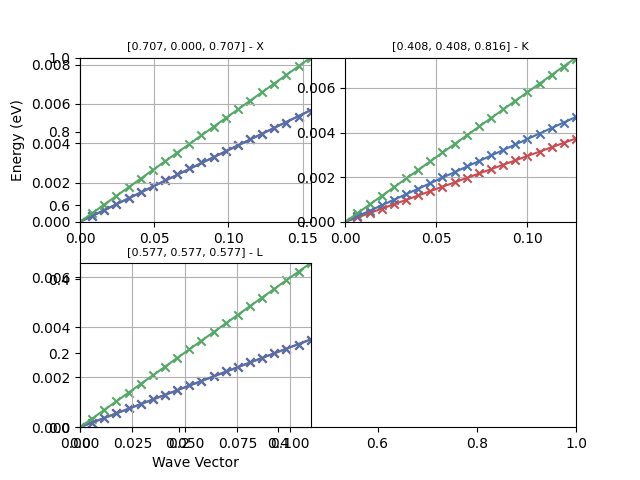# Speed of Sound¶

This example shows how to compute the speed of sound by fitting phonon frequencies along selected directions by linear least-squares fit. For a command line interface use:

abiview.py ddb_vs input_DDBfrom __future__ import print_function

import os
import abipy.data as abidata

from abipy import abilab
from abipy.dfpt.vsound import SoundVelocity

# Initialize object from DDB file.
ddb_path = os.path.join(abidata.dirpath, "refs", "si_sound_vel", "Si_DDB")
sv = SoundVelocity.from_ddb(ddb_path)

# Get pandas dataframe with results.
df = sv.get_dataframe()
abilab.print_dataframe(df)

# Plot fit
sv.plot()
sv.plotly()


Total running time of the script: ( 0 minutes 1.555 seconds)

Gallery generated by Sphinx-Gallery